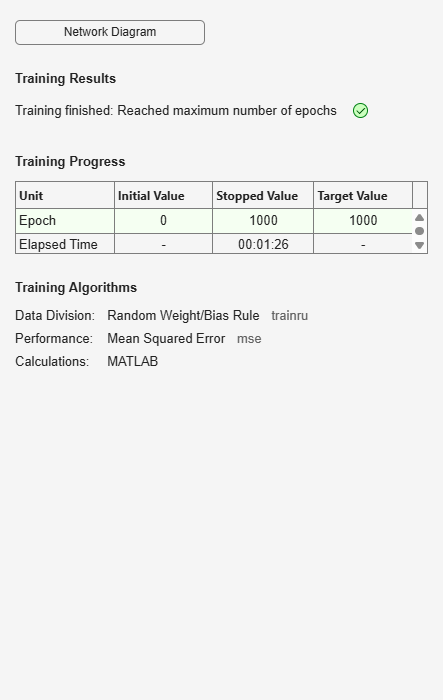competlayer

Competitive layer

Syntax

competlayer(numClasses,kohonenLR,conscienceLR)

Description

Competitive layers learn to classify input vectors into a given number of classes, according to similarity between vectors, with a preference for equal numbers of vectors per class.

competlayer(numClasses,kohonenLR,conscienceLR) takes these arguments,

 numClasses Number of classes to classify inputs (default = 5) kohonenLR Learning rate for Kohonen weights (default = 0.01) conscienceLR Learning rate for conscience bias (default = 0.001)

and returns a competitive layer with numClasses neurons.

Examples

Create and Train a Competitive Layer

Here a competitive layer is trained to classify 150 iris flowers into 6 classes.

inputs = iris_dataset;
net = competlayer(6);
net = train(net,inputs);
view(net)
outputs = net(inputs);
classes = vec2ind(outputs);